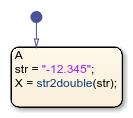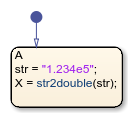# str2double, double

Convert string to double-precision value

Since R2018b

## Syntax

``X = str2double(str)``
``X = double(str)``

## Description

example

````X = str2double(str)` converts the text in string `str` to a double-precision value. In a chart that uses MATLAB® as the action language, `str2double` returns a complex value.In a chart that uses C as the action language, `str2double` returns a real value. If `str2double` cannot convert the text to a number, it returns a `NaN` value.```

example

````X = double(str)` is an alternative way to execute `str2double(str)` in charts that use MATLAB as the action language.```

Note

Stateflow® charts that use C as the action language support calling `double` only with numeric arguments.

## Examples

expand all

Convert the string `"-12.345"` to a double-precision numeric value.

```str = "-12.345"; X = str2double(str);```Alternatively, in charts that use MATLAB as the action language, you can use the operator `double`:

```str = "-12.345"; X = double(str);```Return a value of `123400`.

```str = "1.234e5"; X = str2double(str);```Alternatively, in charts that use MATLAB as the action language, you can use the operator `double`:

```str = "1.234e5"; X = str2double(str);```## Input Arguments

expand all

Input value, specified as a string scalar.

`str` must contain text that represents a number, including:

• Digits

• A decimal point

• A leading `+` or `-` sign

• An `e` preceding a power of 10 scale factor

• An imaginary part followed by an `i` or a `j` (not supported in charts that use C as the action language)

In charts that use MATLAB as the action language, enclose literal strings with double quotes.

## Version History

Introduced in R2018b